## Tangential Method Calculation

The tangential method is the simplest calculation among other directional survey calculations.The tangential method formulas are listed below;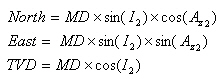Tangential Method Calculation formula

## Minimum Curvature Method

The Minimum Curvature Method smooths two straight-line segments of the Balanced Tangential Method by using the Ratio Factor (RF).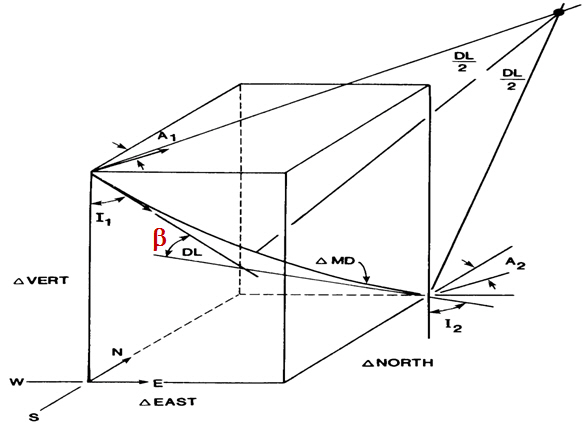The formulas for the Minimum Curvature Method are listed below;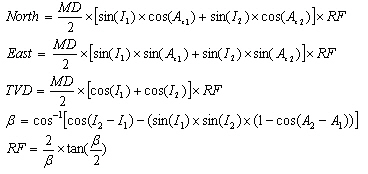## Balanced Tangential Method Calculation

I have some directional calculations that I’ve shared with you. However, some of them are not shared yet so I will try to share all directional drilling formulas that may be helpful for you in the future.

Today, I would like to show you about Balanced Tangential Method Calculation. This calculation method treats half of the measured distance (MD/2) as being tangent to I1 and Az1 and the remainder of the measured distance (MD/2) as being tangent to I2 and Az2.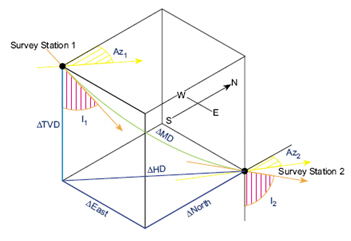## What does the negative vertical section mean?

Vertical Section is the horizontal distance of wellbore that moves in the direction of the target per each station or in total.  For instance, in the figure below, vertical section is the distance from survey to survey point and it’s measured in the same direction of the vertical section direction.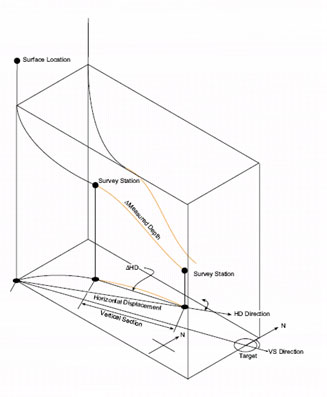The two factors that affect vertical section are as follows:

1. The Incremental horizontal displacement (? HD)

2. Vertical section direction (VSD) is the azimuth that is used to reference to the vertical section. Normally, VSD is the azimuth of the last target.

The simple mathematics as Average Angle Method calculation demonstrates the relationship of the VS as below:

VS = cos (VSD – Az avg) X ?HD

VS: Vertical Section

VSD: Vertical Section Direction

Az avg: Average Azimuth between 2 points (Az1 + Az2) ÷2

?HD: Delta Horizontal Displacement

In order to get the Positive Vertical Section or Zero Vertical Section, a well path must have difference of angle between VSD and Az avg, (VSD – Az avg), within a range of +90 to -90 degree. On the other hands, the negative Vertical Section can occur because the difference of angle between VSD and A zavg, (VSD – Az avg), is out of range of +90 to -90 degree AZI.

## Dogleg Severity Calculation based on Radius of Curvature Method

Dogleg severity (DLS) is a normalized estimation, normally described in degrees per 100 feet or degree per 30 meters, of the overall well bore curvature between two consecutive directional surveys. Regarding a planned well path, dogleg severity may be synonymous about build and/or turn. The following formula provides dogleg severity in degrees/100 ft  based on the Radius of Curvature Method.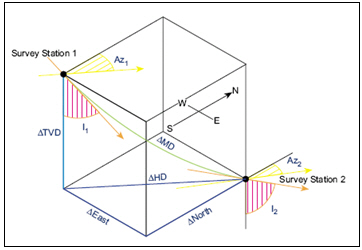Dogleg severity (DLS) = {cos-1 [(cos I1 x cos I2) + (sin I1 x sin I2) x cos (Az2 – Az1)]} x (100 ÷ MD)

Where;

DLS = dogleg severity in degrees/l00 ft

MD = Measured Depth between survey points in ft

I1 = Inclination (angle) at upper survey in degrees

I2 = Inclination (angle) at lower in degrees

Az1= Azimuth direction at upper survey

Az2 = Azimuth direction at lower survey

Example for dogleg severity based on Radius of Curvature Method

Survey 1

Depth = 7500 ft

Inclination = 45 degree (I1)

Azimuth = 130degree (Az1)

Survey 2

Depth = 7595 ft

Inclination = 52 degree (I2)

Azimuth = 139 degree (Az2)

Dogleg severity (DLS) = {cos-1 [(cos 45 x cos 52) + (sin 45 x sin 52) x cos (139 – 130)]} x (100 ÷ 95)

Dogleg severity (DLS) =  10.22 degree/100 ft

Please find the Excel sheet used to calculate dogleg severity based on Radius of Curvature Method.